﻿ Weierstrass逼近定理在回归分析建模中的应用 Applications of Weierstrass Theorem in Regression Analysis and Mathematical Modeling

Modeling and Simulation
Vol.05 No.04(2016), Article ID:18919,5 pages
10.12677/MOS.2016.54016

Applications of Weierstrass Theorem in Regression Analysis and Mathematical Modeling

Xiao Yu*, Zhan Li

School of Communication and Information Engineering, University of Electronic Science and Technology of China, Chengdu Sichuan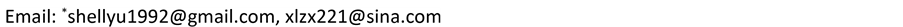Received: Oct. 19th, 2016; accepted: Nov. 8th, 2016; published: Nov. 11th, 2016ABSTRACT

Based on Weierstrass’ approximation theorem, the mathematical principle of a nonlinear regression model which can be approximated by a polynomial regression model is interpreted, and then the general method of converting the multivariate nonlinear regression analysis into the multivariate linear regression analysis is introduced. To show the practicability and validity, a practical application example is given.

Keywords:Weierstrass’ Approximation Theorem, Regression Analysis, Mathematical Model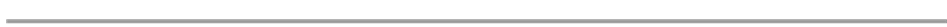Weierstrass逼近定理在回归分析建模中的应用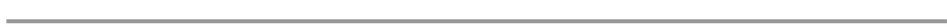1. 引言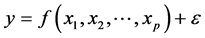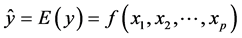.

2. Weierstrass逼近定理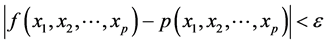.

3. 多元多项式回归模型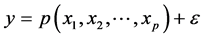4. 应用案例分析

4.1. 问题：农作物施肥效果分析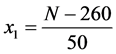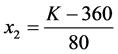4.2. 失败的尝试：线性回归模型,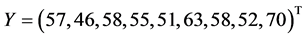.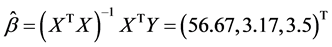..

4.3. 有效的模型：多项式回归模型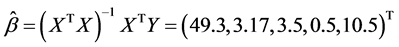.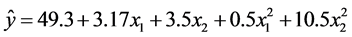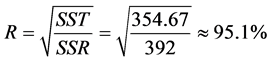.

4.4. 完善的模型：含交叉项的多项式回归模型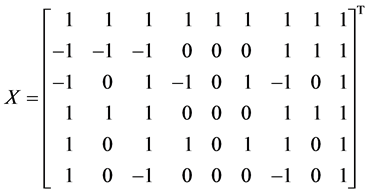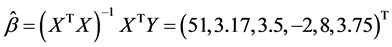.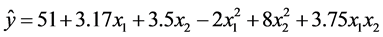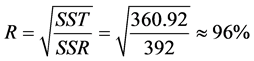.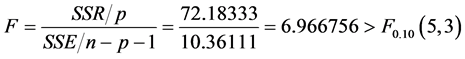5. 结束语

Applications of Weierstrass Theorem in Regression Analysis and Mathematical Modeling[J]. 建模与仿真, 2016, 05(04): 118-122. http://dx.doi.org/10.12677/MOS.2016.54016

1. 1. Rudin, W. (1976) Principles of Mathematical Analysis. McGraw-Hill Companies, Inc., New York.

2. 2. 白其峥. 数学建模案例分析[M]. 北京: 海洋出版社, 2000.

3. 3. 邓力, 范庚, 刘治学. 基于回归分析方法的网络故障预测[J]. 计算机工程, 2012, 38(20): 251-255.

4. 4. 邓如意, 胥义, 王健. 基于多项式回归模型的智能手机图像颜色校正研究[J]. 软件导刊, 2016, 15(1): 173-175.

5. 5. 刘泽春, 李培军. 二次多项曲线拟合在锌测定中的应用[J]. 浙江预防医学, 2015, 27(12): 1294-1296.

6. 6. 李晓明, 刘明, 宋冰燕, 田向荣. 基于三次多项式回归法的大豆种子生活力评价[J]. 吉林大学学报(自然科学版), 2012, 33(5): 83-91.